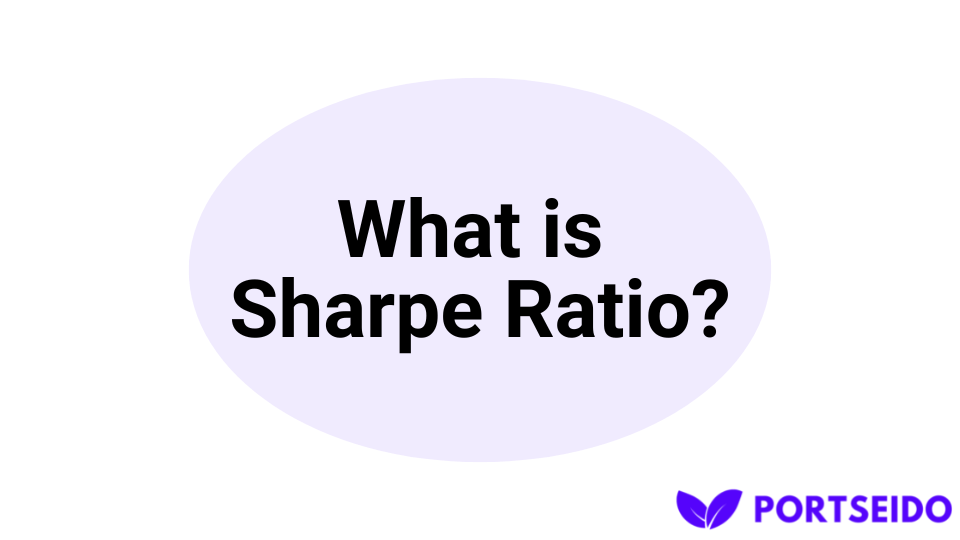Start tracking your investments with Portseido today
Try Portseido for free, and explore all the tools you need to track and plan all your investments.
Home Portseido Blog What is Sharpe Ratio?

# What is Sharpe Ratio?

Article last updated: January 4, 2023The Sharpe Ratio is a risk-adjusted return ratio that compares an investment's return to its risk. It was created in the 1960s by Nobel winner William Sharpe as a means to assess the success of investment portfolios.

## How to calculate Sharpe Ratio?

You'll need three pieces of information to compute the Sharpe Ratio: the investment's return, the risk-free rate, and the standard deviation of the investment's returns.

The Sharpe Ratio is calculated as follows:

``````Sharpe Ratio = (Investment Return - Risk-Free Rate) / Investment Return Standard Deviation
``````

## Example of how to calculate Sharpe Ratio

Assume you have a stock that has returned 10% in the last year and the risk-free rate (such as the yield on a Treasury bond) is 3%. The stock's returns have a standard deviation of 5%. When we enter these figures into the formula, we get:

``````Sharpe Ratio = (5% - 10%) / 5% = 1.4
``````

With a Sharpe Ratio of 1.4, the stock has earned 1.4 times greater return per a unit of risk than the risk-free rate.

## What is a good Sharpe Ratio?

A decent Sharpe Ratio is generally thought to be greater than 1.0, though this can vary depending on the conditions. A greater Sharpe Ratio suggests that the investment delivered a higher amount of return per unit of risk.

## How to use Sharpe Ratio?

It's crucial to remember that the Sharpe Ratio is only one measure of risk-adjusted return, and it should be used with other measures to get a complete picture of an investment's performance. The Sharpe Ratio does not take into consideration certain categories of risk, such as liquidity risk or tail risk, since it uses standard deviation of historical return as risk. This means it assumes that the more volatile the return, the riskier the assets. Furthermore, it is calculated using historical returns rather than future projections.

Finally, the Sharpe Ratio is a valuable measure for determining an investment's risk-adjusted return. It can be used to compare the performance of different assets and helps investors understand how much return they are getting for the level of risk they are taking on.

Want to track all your investments in one place? Start your free 14-day trial of Portseido—no credit card required.